# Maximum of volume

The shell of the cone is formed by winding a circular section with a radius of 1. For what central angle of a given circular section will the volume of the resulting cone be maximum?

A =  293.9388 °

### Step-by-step explanation: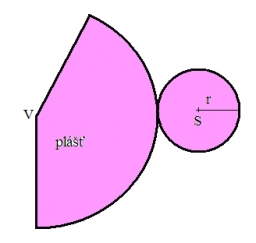Did you find an error or inaccuracy? Feel free to write us. Thank you!Tips to related online calculators
Do you have a linear equation or system of equations and looking for its solution? Or do you have a quadratic equation?
Pythagorean theorem is the base for the right triangle calculator.
Tip: Our volume units converter will help you with the conversion of volume units.

#### You need to know the following knowledge to solve this word math problem:

We encourage you to watch this tutorial video on this math problem:

## Related math problems and questions:

• Circular sector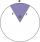I have a circular sector with a length 15 cm with an unknown central angle. It is inscribed by a circle with radius 5 cm. What is the central angle alpha in the circular sector?
• Cone A2VThe surface of the cone in the plane is a circular arc with central angle of 126° and area 415 cm2. Calculate the volume of a cone.
• Cut and coneCalculate the volume of the rotation cone which lateral surface is circle arc with radius 15 cm and central angle 63 degrees.
• Circle sectionEquilateral triangle with side 33 is inscribed circle section whose center is in one of the vertices of the triangle and the arc touches the opposite side. Calculate: a) the length of the arc b) the ratio betewwn the circumference to the circle sector and
• Circle sectorThe circular sector with a central angle 160° has an area 452 cm2. Calculate its radius r.
• Circular segmentCalculate the area S of the circular segment and the length of the circular arc l. The height of the circular segment is 2 cm and the angle α = 60°. Help formula: S = 1/2 r2. (Β-sinβ)
• Sphere and coneWithin the sphere of radius G = 33 cm inscribe the cone with the largest volume. What is that volume, and what are the dimensions of the cone?
• Martians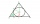A sphere-shaped spaceship with a diameter of 6 m landed in the meadow. In order not to attract attention, the Martians covered it with a roof in the shape of a regular cone. How high will this roof be so that the consumption of roofing is minimal?
• Circular poolThe base of the pool is a circle with a radius r = 10 m, excluding a circular segment that determines the chord length 10 meters. The pool depth is h = 2m. How many hectoliters of water can fit into the pool?
• SectorThe perimeter of a circular sector with an angle 1.8 rad is 64 cm. Determine the radius of the circle from which the sector comes.
• The central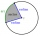The central angle of a sector is 30° and the radius is 15 m. Determine its perimeter.
• Volume of the coneCalculate the volume of the cone if the content of its base is 78.5 cm2 and the content of the shell is 219.8 cm2.
• Axial sectionThe axial section of the cone is an equilateral triangle with area 168 cm2. Calculate the volume of the cone.
• Right circular coneThe volume of a right circular cone is 5 liters. Calculate the volume of the two parts into which the cone is divided by a plane parallel to the base, one-third of the way down from the vertex to the base.
• Circle arcCircle segment has a circumference of 135.26 dm and 2096.58 dm2 area. Calculate the radius of the circle and size of the central angle.
• Axial sectionAxial section of the cylinder has a diagonal 40 cm. The size of the shell and the base surface are in the ratio 3:2. Calculate the volume and surface area of this cylinder.
• Arc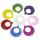What area of a circle occupied the flowers planted in the arc of a circle with radius 3 m with central angle 45°?